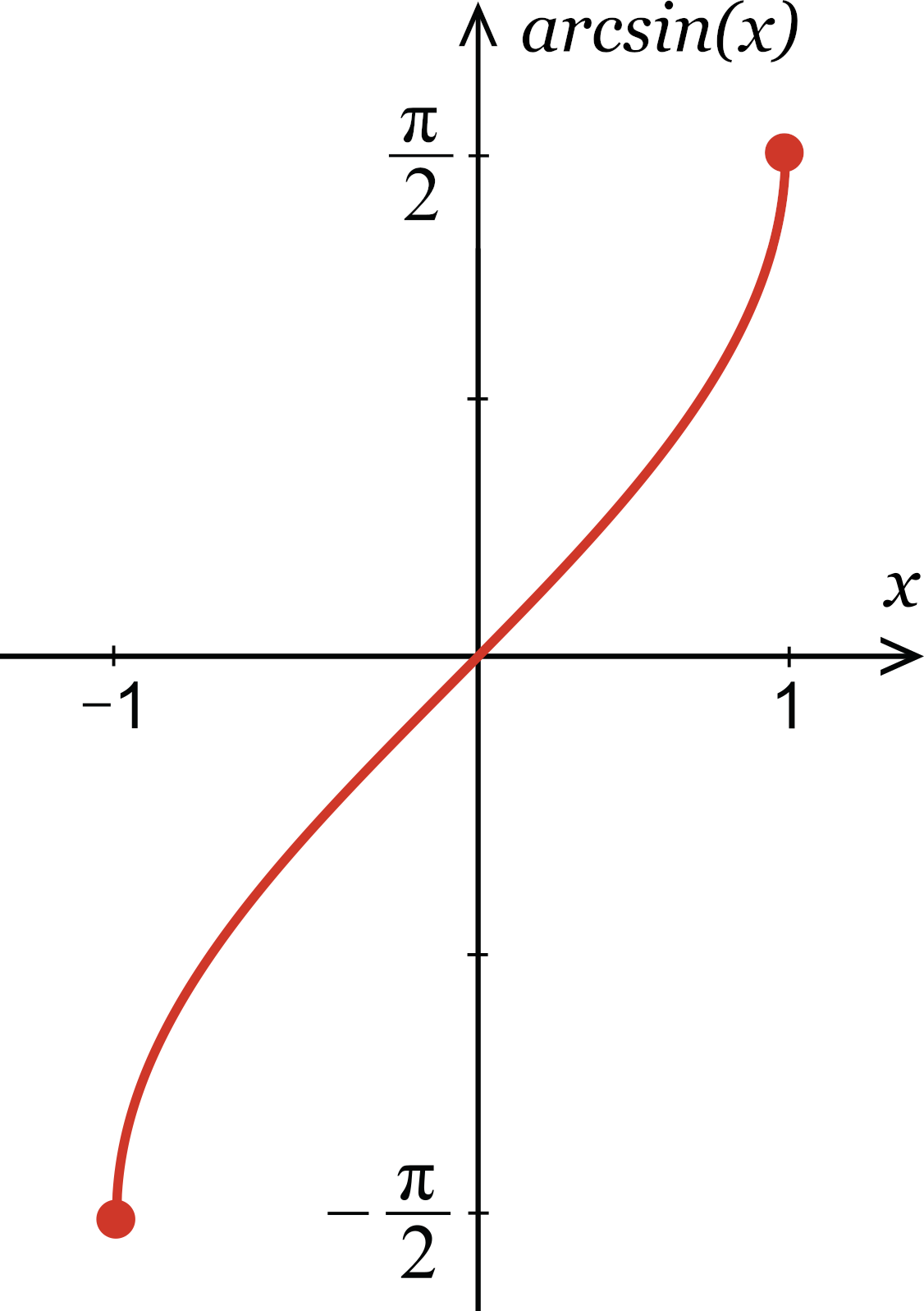# Inverse Sine Calculator – Calculate arcsin(x)

Find the angle in degrees or radians using the inverse sine with the arcsin calculator below.

## How to Find Arcsin

Arcsin is a trigonometric function to calculate the inverse sine. Arcsin can also be expressed as sin-1(x).

The term inverse means the opposite or to “undo” something. For example, multiplication and division or inverse operations.

Arcsin is used to undo or reverse the sine function. If you know the sine of an angle, you can use arcsin to calculate the measurement of an angle.

Since arcsin is the inverse of the sine function, and many angles share the same sine value, arcsin is a periodic function. Each arcsin value can result in multiple angle values, which is why the domain is restricted to [-1, 1].

The primary result for arcsin is known as the principal value and is the angle in the range of -90° to 90°.

To calculate arcsin, use a scientific calculator and the asin or sin-1 function, or just use the calculator above. Most scientific calculators require the angle value in radians to solve for sin.

### Inverse Sine Formula

The inverse sine formula is:

y = sin(x) | x = arcsin(y)

Thus, if y is equal to the sine of x, then x is equal to the arcsin of y.

## Inverse Sine Graph

If you graph the arcsin function for every possible value of sine, it forms an increasing curve from (-1, -π/2) to (1, π/2).Because the value of the sine function oscillates in the range of -1 to 1, the inverse sine curve’s domain starts at x = -1 and ends at x = 1. Since the peak (maximum) of the sine wave is at π/2 radians, and the dip (minimum) of the wave is at -π/2 radians, the y value ends at those points.

## Inverse Sine Table

The table below shows common sine values and the arcsin, or angle, for each of them.

Table showing common sine values and inverse sine values for each in degrees and radians.
-1 -90° π / 2
√6 + √2 / 4 -75° / 12
√3 / 2 -60° π / 3
√2 / 2 -45° π / 4
1 / 2 -30° π / 6
√6 – √2 / 4 -15° π / 12
0 0
√6 – √2 / 4 15° π / 12
1 / 2 30° π / 6
√2 / 2 45° π / 4
√3 / 2 60° π / 3
√6 + √2 / 4 75° / 12
1 90° π / 2

You might also be interested in our inverse cosine and inverse tangent calculators.

## How to Use Inverse Sine to Find an Angle in a Right Triangle

You can find the angle in a right triangle by finding the arcsin.

Begin by identifying and labeling the hypotenuse, opposite side, and adjacent side in regards to the angle you want to find.

Use the equation y = arcsin(opposite/hypotenuse) and evaluate.

If the opposite side and hypotenuse are known, you can find the value of y directly and round the answer to the nearest degree or decimal place.

If the opposite side and hypotenuse are not known, you can use the Pythagorean theorem to find the missing side lengths before using the above formula.

## The Relationship Between Arcsin and Arccos

Arcsine and arccosine are complementary to one another due to the fact that the arcsine function gives us the angle whose sine is a given ratio. This can be seen with the expression: ​​arcsin(x) = π/2 – arccos(x).

The arcsin function gives us the angle whose sine is a given ratio, while the arccos function gives us the angle whose cosine is a given ratio. These angles are different in a right triangle, but they are always complementary, meaning that their sum is always 90 degrees (or π/2 radians).

### What is sine to the power of -1?

Sine-1 refers to the inverse sine function or arcsine. This function takes a value between -1 and 1 as the input and returns an angle in radians as the output.

For example, if sin(x) = -0.866, then sin-1(-0.866) = -1.047 radians. This is approximately -60 degrees which means that the angle whose sine is -0.866 is -60 degrees or -1.047 radians.

### Can you find the inverse sine without a calculator?

Yes, you can find the inverse sine, or arcsine, without a calculator by identifying the value that you want to find the inverse sine for. Then write down the equation ​​sin(y) = x and solve for y by taking the arcsine of both sides of the equation.

You can then evaluate the expression using algebraic methods for simple fractions or geometric methods for more complex values. Some values, however, may require you to use the table of trigonometric values.

### Can you find the inverse sine for an angle in degrees?

Yes, once you find arcsin for an angle in radians, you can convert the value to degrees with the formula ​​radians = (π / 180) × degrees or using our degrees to radians calculator.

### Is the inverse sine the same as 1 over sin?

Although this is a common mistake, inverse sine is not the same as 1/sine. Arcsine is the inverse of the sine function where 1/sine is the reciprocal of the sine.

### Can arcsine be negative?

Yes, arcsine can be negative. A negative arcsine is the inverse or arcsine of a negative value [arcsin(-x) = -arcsin(x)]. A negative sign represents the direction of the angle.

This means that a negative angle represents a rotation in the opposite direction from the positive angle with the same magnitude.